CDA数据科学研究院 CDA考试中心 企业服务 关于CDA

#全国校区## 豆瓣9.2！17万条弹幕告诉你《沉默的真相》凭什么高口碑！2020-09-27CDA数据分析师 出品

【导读】今天教大家用Python分析《沉默的真相》的17万条弹幕。距离上一部国产良心剧《隐秘的角落》刷屏还不到2个月，“秃头梗”、“爬山梗”还让人记忆犹新。紧接着又一部爆款国产剧来了，那就是最近口碑炸裂的《沉默的真相》。同样是来自爱奇艺针对悬疑短剧的“迷雾剧场”，《沉默的真相》根据紫金陈的小说《长夜难明》改编，讲述了检察官江阳历经多年，付出无数代价查清案件真相的故事。01、豆瓣 9.2分！超越前浪《隐秘的角落》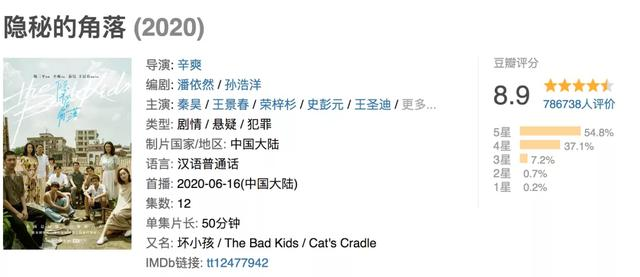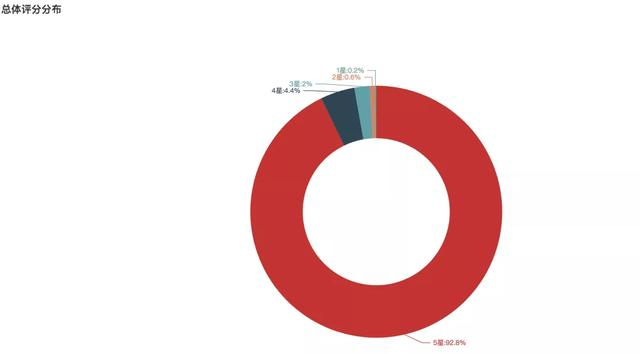92.8%的观众给出了五星满分，这口碑在国产剧中已经达到标杆的水准。02、刷剧《沉默的真相》，17万条弹幕都在说些什么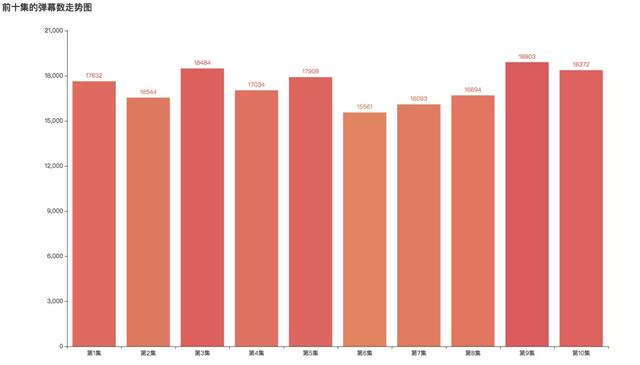李静弹幕词云03、手把手教你，如何用Python分析弹幕

1. 弹幕数据获取
2. 数据读入和简单处理
3. 数据可视化分析

1. 数据获取

2. 数据读入和预处理

```# 导入库
import os
import jieba
import pandas as pd

from pyecharts.charts import Bar, Pie, Line, WordCloud, Page
from pyecharts import options as opts
from pyecharts.globals import SymbolType, WarningType
WarningType.ShowWarning = False

import stylecloud
from IPython.display import Image```

```# 读入数据
data_list = os.listdir('../data/')

df_all = pd.DataFrame()

for i in data_list:
if i.endswith('csv'):
df_one = pd.read_csv(f'../data/{i}', engine='python', encoding='utf-8', index_col=0)
df_all = df_all.append(df_one, ignore_index=False)

print(df_all.shape) ```
`(173226, 6)`

```df_all['name'] = df_all.name.str.strip()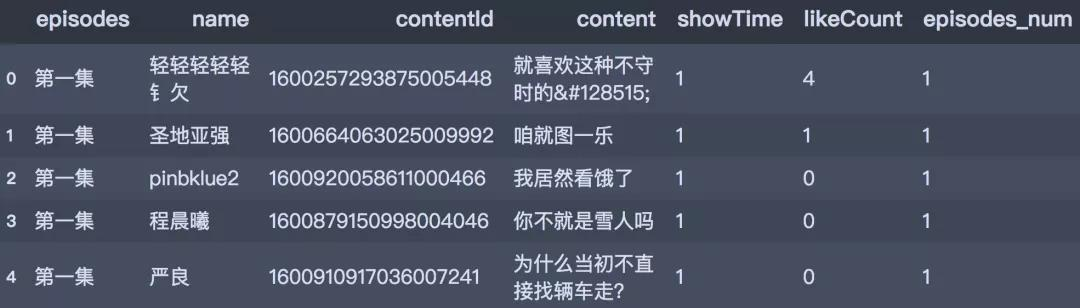3. 数据可视化

——分集的弹幕数```repl_list = {
'第一集 ': 1,
'第二集': 2,
'第三集': 3,
'第四集': 4,
'第五集': 5,
'第六集': 6,
'第七集': 7,
'第八集': 8,
'第九集': 9,
'第十集': 10
}

df_all['episodes_num'] = df_all['episodes'].map(repl_list)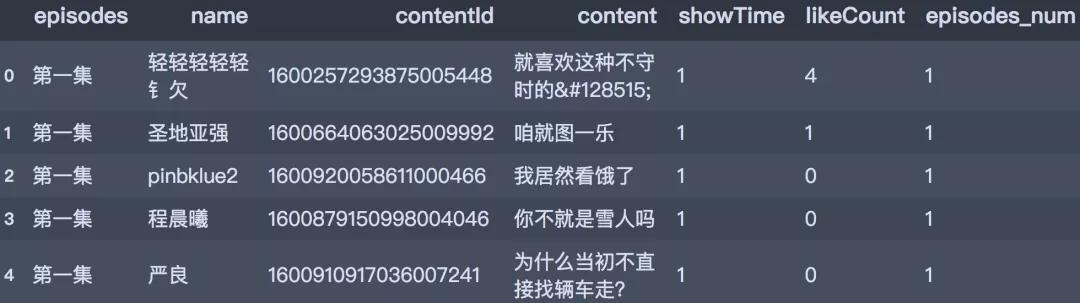```# 产生数据
danmu_num = df_all.episodes_num.value_counts()
danmu_num = danmu_num.sort_index()
x_data = ['第' + str(i) + '集' for i in danmu_num.index]
y_data = danmu_num.values.tolist()

# 条形图
bar1 = Bar(init_opts=opts.InitOpts(width='1350px', height='750px'))
bar1.set_global_opts(title_opts=opts.TitleOpts(title='前十集的弹幕数走势图'),
visualmap_opts=opts.VisualMapOpts(max_=20000, is_show=False)
)
bar1.render() ```
```x_data = ['第' + str(i) + '集' for i in danmu_num.index]
y_data = danmu_num.values.tolist()

# 条形图
bar1 = Bar(init_opts=opts.InitOpts(width='1350px', height='750px'))
bar1.set_global_opts(title_opts=opts.TitleOpts(title='前十集的弹幕数走势图'),
visualmap_opts=opts.VisualMapOpts(max_=20000, is_show=False)
)
bar1.render('../html/爱奇艺弹幕数走势图.html') ``````# 定义分词函数
def get_cut_words(content_series):
# 读入停用词表
stop_words = []

with open(r"stop_words.txt", 'r', encoding='utf-8') as f:
for line in lines:
stop_words.append(line.strip())

# 添加关键词
my_words = ['廖凡', '严良', '白宇', '江阳', '谭卓', '李静',
'宁理', '张超', '黄尧', '张晓倩', '奥利给'
]

for i in my_words:

# 自定义停用词
my_stop_words = ['真的', '这部', '这是', '一种', '那种', '啊啊啊', '哈哈哈',
'哈哈哈哈', '我要']
stop_words.extend(my_stop_words)

# 分词
word_num = jieba.lcut(content_series.str.cat(sep='。'), cut_all=False)

# 条件筛选
word_num_selected = [i for i in word_num if i not in stop_words and len(i)>=2]

return word_num_selected```
```# 获取分词结果
text1 = get_cut_words(content_series=df_all[df_all.name=='江阳']['content'])

# 绘制词云图
stylecloud.gen_stylecloud(text=' '.join(text1), max_words=1000,
collocations=False,
font_path=r'‪C:\Windows\Fonts\msyh.ttc',
icon_name='fas fa-heart',
size=653,
output_name='弹幕角色-江阳词云图.png') ```

OK